# RS Aggarwal Solutions for Class 10 Maths Chapter 1 Real Numbers Exercise 1E Page number 34

RS Aggarwal Solutions for Class 10 Maths Chapter 1 Real Numbers, contains solutions for all Exercise 1E questions. Students can practice on real number concepts especially Euclid’s Division Lemma, its applications, fundamental theorem, rational Numbers and irrational Numbers. Download the RS Aggarwal Solutions of Class 10 now and improve your scores.

### Download PDF of RS Aggarwal Solutions for Class 10 Maths Chapter 1 Real Numbers Exercise 1E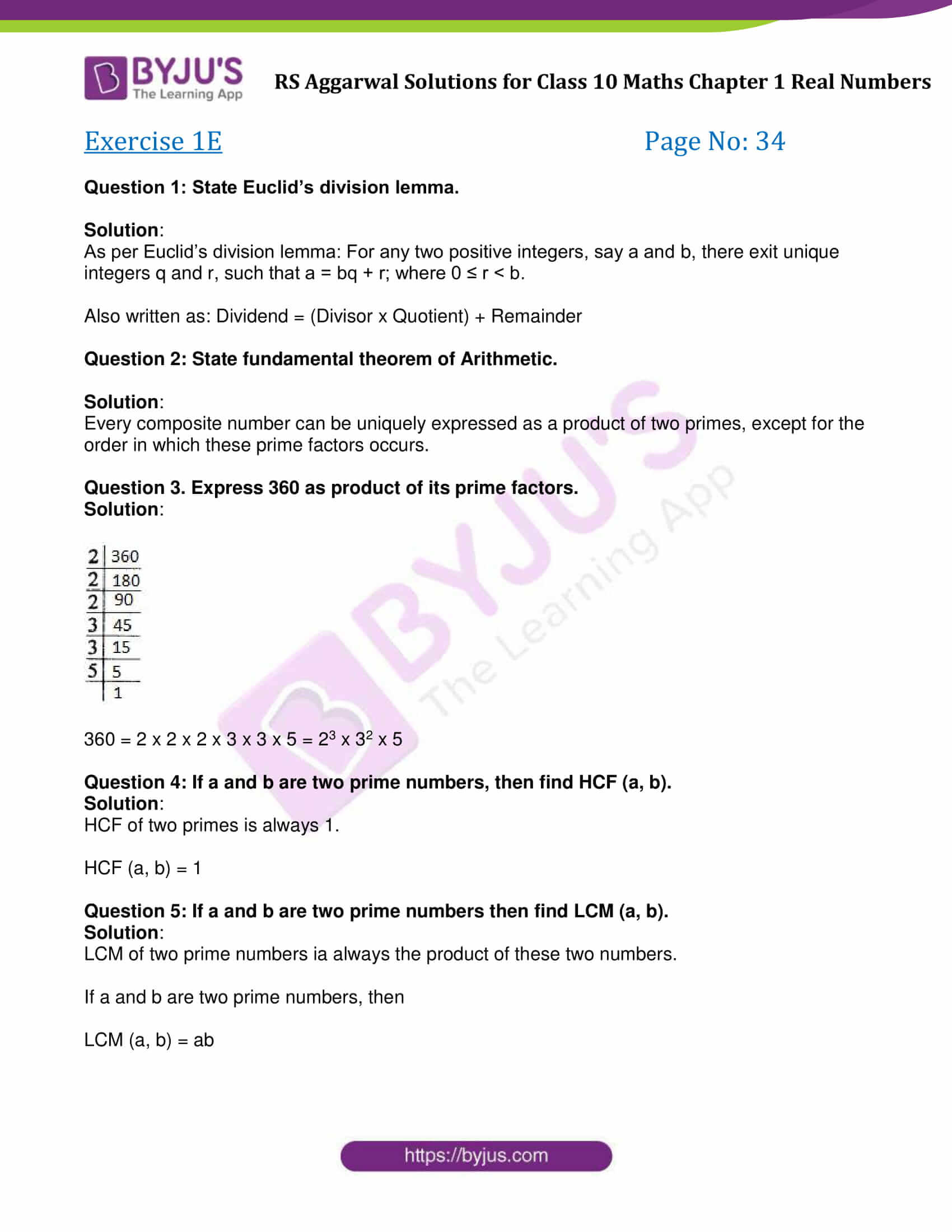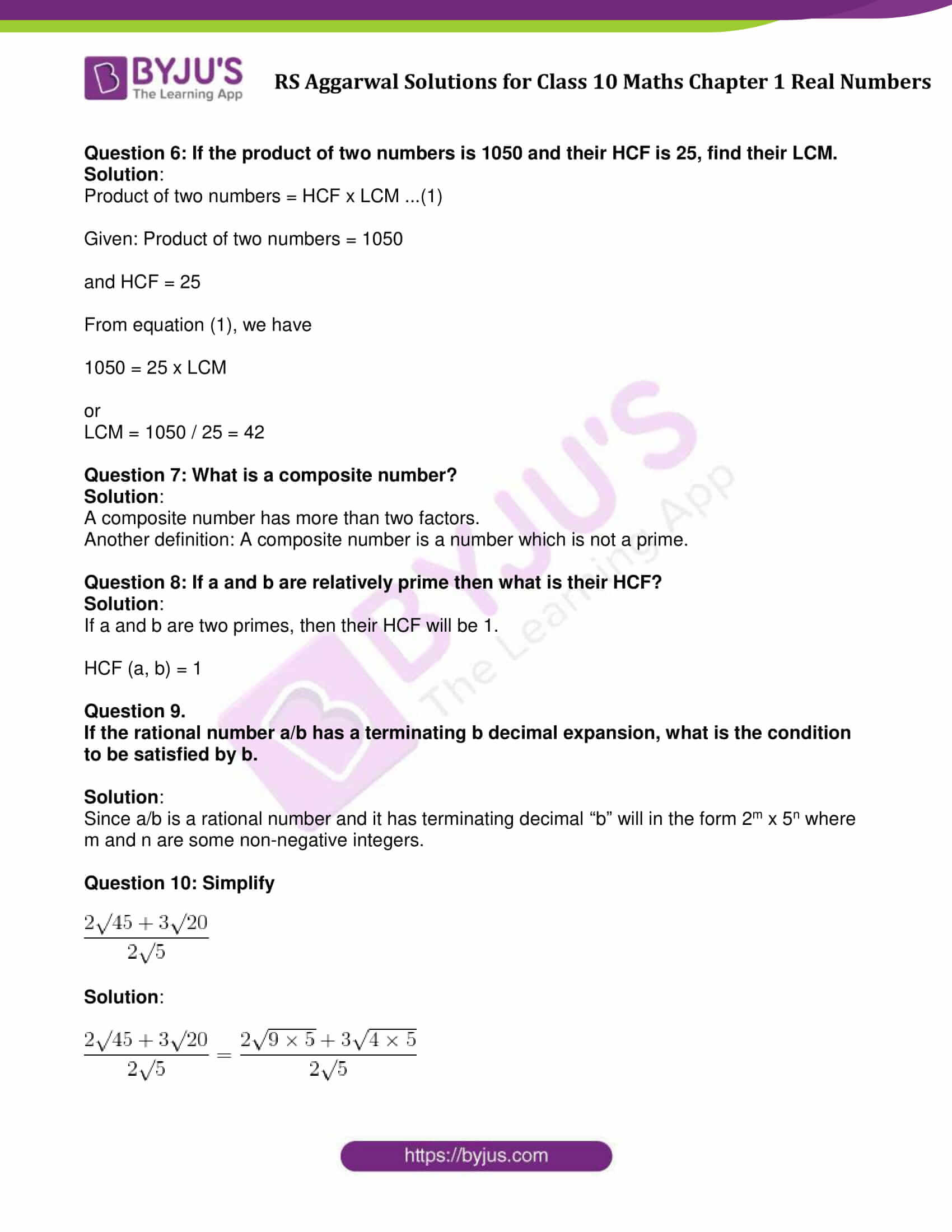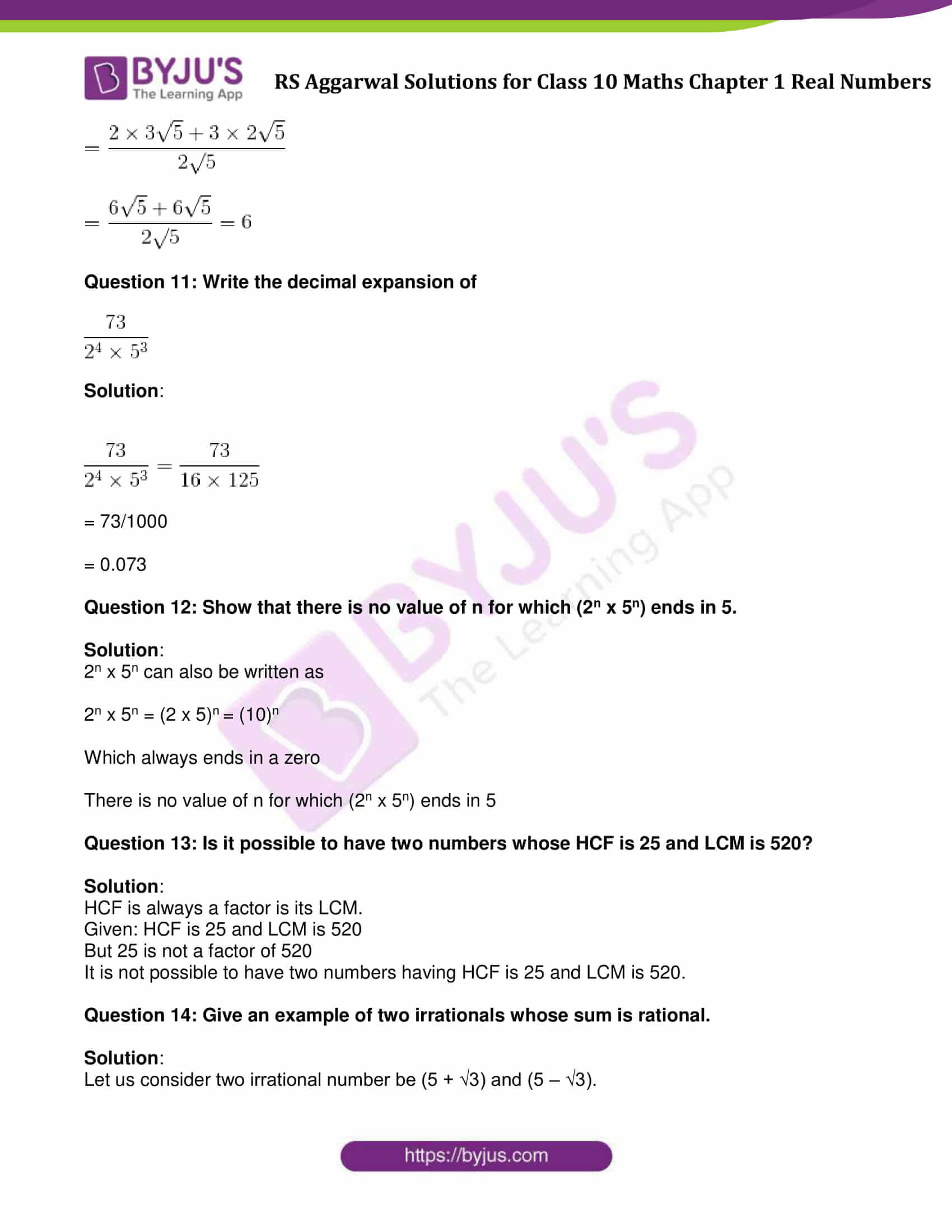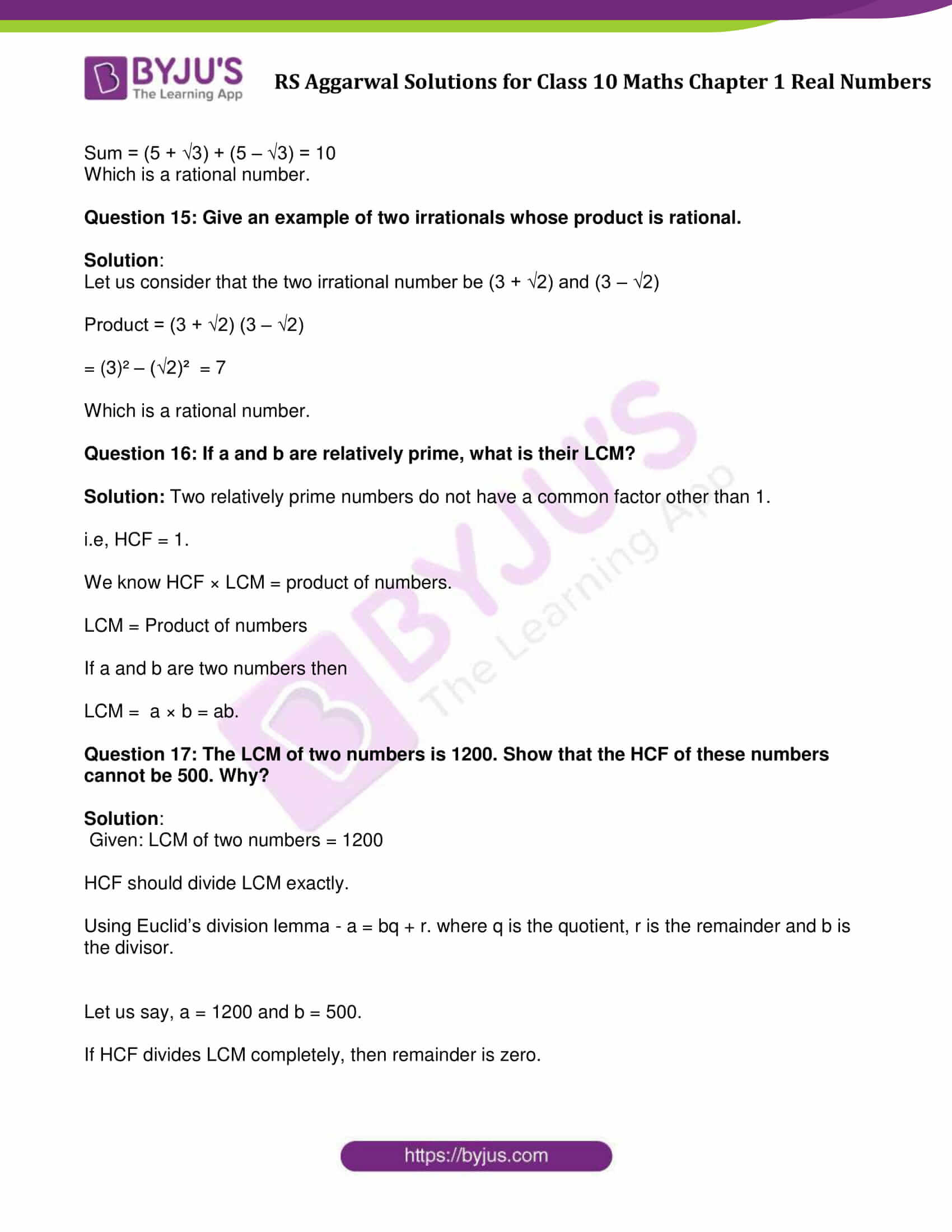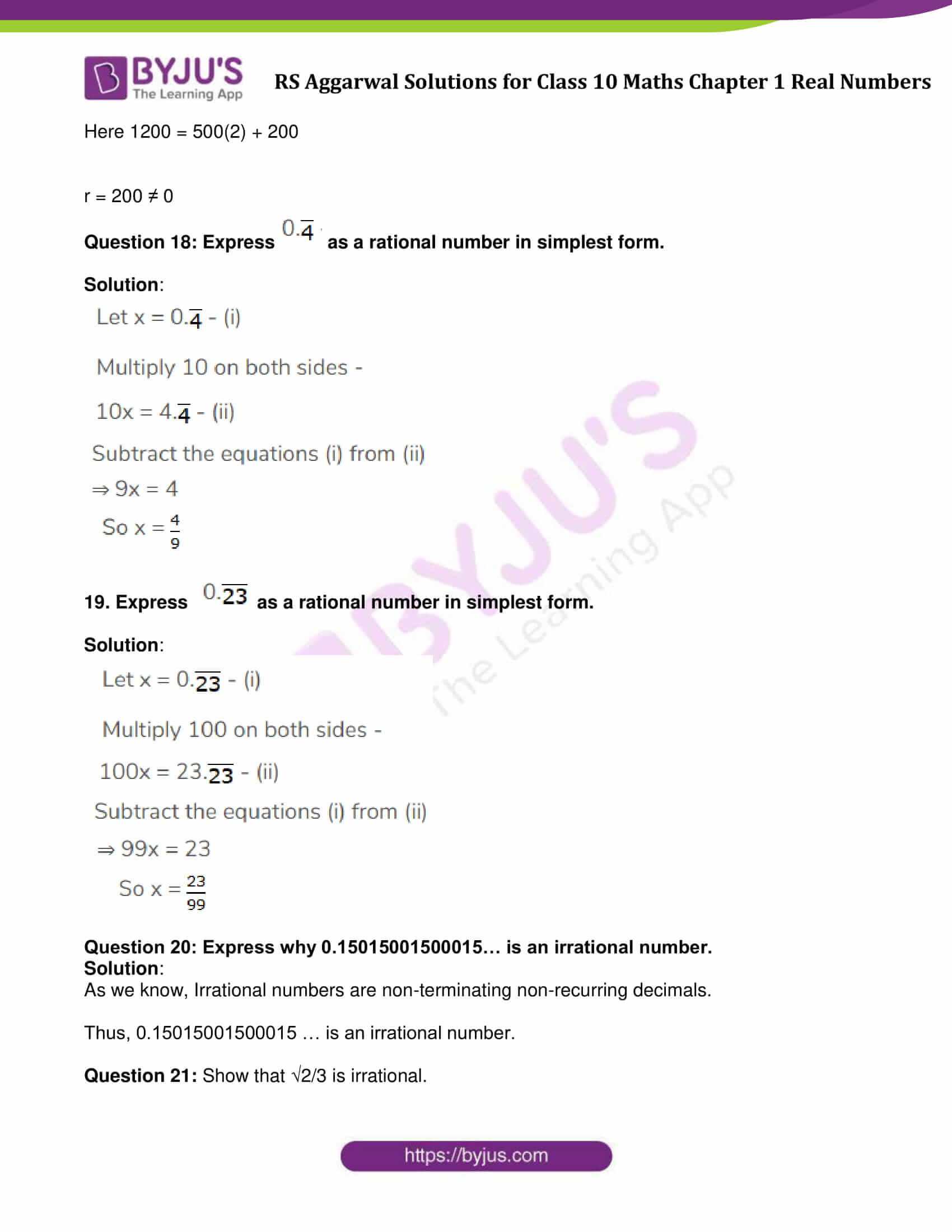### Access other exercise solutions of Class 10 Maths Chapter 1 Real Numbers

Exercise 1A Solutions: 10 Questions (Short Answers)

Exercise 1B Solutions: 27 Questions (Short Answers)

Exercise 1C Solutions: 3 Questions (Short Answers)

Exercise 1D Solutions: 11 Questions (Short Answers)

## Exercise 1E Page No: 34

Question 1: State Euclid’s division lemma.

Solution:

As per Euclid’s division lemma: For any two positive integers, say a and b, there exit unique integers q and r, such that a = bq + r; where 0 ≤ r < b.

Also written as: Dividend = (Divisor x Quotient) + Remainder

Question 2: State fundamental theorem of Arithmetic.

Solution:

Every composite number can be uniquely expressed as a product of two primes, except for the order in which these prime factors occurs.

Question 3. Express 360 as product of its prime factors.

Solution: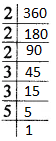360 = 2 x 2 x 2 x 3 x 3 x 5 = 23 x 32 x 5

Question 4: If a and b are two prime numbers, then find HCF (a, b).

Solution:

HCF of two primes is always 1.

HCF (a, b) = 1

Question 5: If a and b are two prime numbers then find LCM (a, b).

Solution:

LCM of two prime numbers ia always the product of these two numbers.

If a and b are two prime numbers, then

LCM (a, b) = ab

Question 6: If the product of two numbers is 1050 and their HCF is 25, find their LCM.

Solution:

Product of two numbers = HCF x LCM …(1)

Given: Product of two numbers = 1050

and HCF = 25

From equation (1), we have

1050 = 25 x LCM

or

LCM = 1050 / 25 = 42

Question 7: What is a composite number?

Solution:

A composite number has more than two factors.

Another definition: A composite number is a number which is not a prime.

Question 8: If a and b are relatively prime then what is their HCF?

Solution:

If a and b are two primes, then their HCF will be 1.

HCF (a, b) = 1

Question 9.

If the rational number a/b has a terminating b decimal expansion, what is the condition to be satisfied by b.

Solution:

Since a/b is a rational number and it has terminating decimal “b” will in the form 2m x 5n where m and n are some non-negative integers.

Question 10: Simplify

$\frac{2\sqrt{45} + 3\sqrt{20}}{2\sqrt{5}}$

Solution:

$\frac{2\sqrt{45} + 3\sqrt{20}}{2\sqrt{5}}$ = $\frac{2\sqrt{9\times5} + 3\sqrt{4\times5}}{2\sqrt{5}}$ $\frac{2\times3\sqrt{5} + 3\times2\sqrt{5}}{2\sqrt{5}}$ $\frac{6\sqrt{5} + 6\sqrt{5}}{2\sqrt{5}}$

= 6

Question 11: Write the decimal expansion of

$\frac{73}{2^{4}\times5^{3}}$

Solution:

$\frac{73}{2^{4}\times5^{3}}$ = $\frac{73}{16\times125}$

= 73/1000

= 0.073

Question 12: Show that there is no value of n for which (2n x 5n) ends in 5.

Solution:

2n x 5n can also be written as

2n x 5n = (2 x 5)n = (10)n

Which always ends in a zero

There is no value of n for which (2n x 5n) ends in 5

Question 13: Is it possible to have two numbers whose HCF is 25 and LCM is 520?

Solution:

HCF is always a factor is its LCM.

Given: HCF is 25 and LCM is 520

But 25 is not a factor of 520

It is not possible to have two numbers having HCF is 25 and LCM is 520.

Question 14: Give an example of two irrationals whose sum is rational.

Solution:

Let us consider two irrational number be (5 + √3) and (5 – √3).

Sum = (5 + √3) + (5 – √3) = 10

Which is a rational number.

Question 15: Give an example of two irrationals whose product is rational.

Solution:

Let us consider that the two irrational number be (3 + √2) and (3 – √2)

Product = (3 + √2) (3 – √2)

= (3)² – (√2)² = 7

Which is a rational number.

Question 16: If a and b are relatively prime, what is their LCM?

Solution: Two relatively prime numbers do not have a common factor other than 1.

i.e, HCF = 1.

We know HCF × LCM = product of numbers.

LCM = Product of numbers

If a and b are two numbers then

LCM = a × b = ab.

Question 17: The LCM of two numbers is 1200. Show that the HCF of these numbers cannot be 500. Why?

Solution:

Given: LCM of two numbers = 1200

HCF should divide LCM exactly.

Using Euclid’s division lemma – a = bq + r. where q is the quotient, r is the remainder and b is the divisor.

Let us say, a = 1200 and b = 500.

If HCF divides LCM completely, then remainder is zero.

Here 1200 = 500(2) + 200

r = 200 ≠ 0

Question 18: Express $0.\overline{4}$ as a rational number in simplest form.

Solution: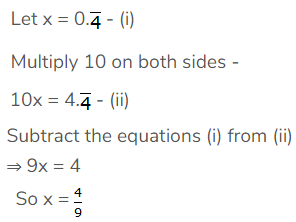19. Express $0.\overline{23}$ as a rational number in simplest form.

Solution: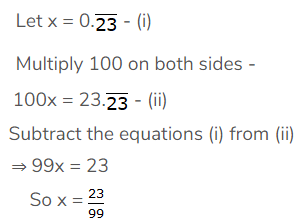Question 20: Express why 0.15015001500015… is an irrational number.

Solution:

As we know, Irrational numbers are non-terminating non-recurring decimals.

Thus, 0.15015001500015 … is an irrational number.

Question 21: Show that √2/3 is irrational.

Solution: Let us assume that √2/3 is rational

Which is possible if 1/3 is rational and √2 is rational

But the fact is √2 is an irrational.

Which is contradict, our assumption is wrong.

√2/3 is irrational.

Question 22: Write a rational number between √3 and 2.

Solution:

The value of √3 is 1.73.

A rational number between √3 and 2 is 1.8.

Question 23: Explain why $3.\overline{1416}$is a rational number.

Solution:

$3.\overline{1416}$ is a non – terminating repeating decimal.

So it is a rational number.

## RS Aggarwal Solutions for Class 10 Maths Chapter 1 Real Numbers Exercise 1E

Class 10 Maths Chapter 1 Real Numbers Exercise 1E, based on the following topics and subtopics:

• Euclid’s Division Lemma
• Some Applications of Euclid’s Division Lemma
• Fundamental Theorem of Arithmetic
• Rational Numbers
• Irrational Numbers
• Important results on Irrational